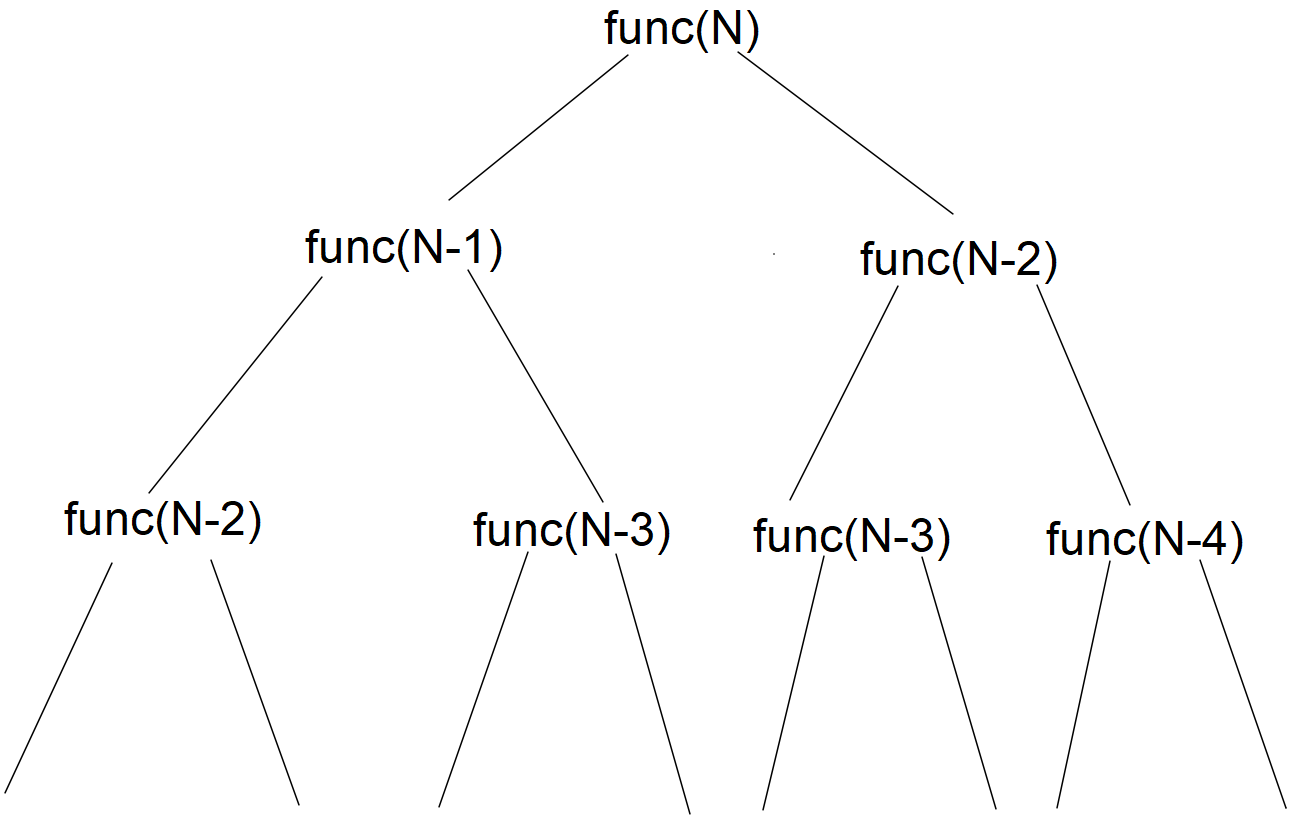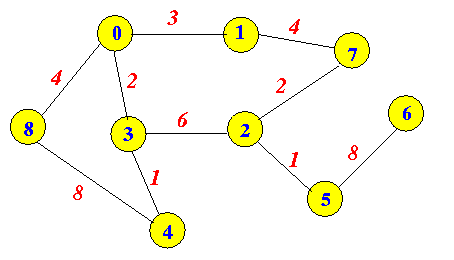# Overlapping substructure vs overlapping sub problems

## Introduction:

Before jumping to Optimal substructure and Overlapping subproblems, let us first see what does dynamic programming mean?

Dynamic programming is a technique we apply to recursive problems. Suppose in any recursive problem we compute the answer for the same subproblem multiple times. In that case, we can store the answer for that subproblem to save the time of re-calculating the same subproblem, which helps us to bring down the exponential time complexity to the polynomial-time complexity. Hence, to apply dynamic programming, recursive problems should have Optimal substructure or Overlapping subproblems, which we will discuss further.

## Overlapping subproblems

As we know when we solve the recursive problems, we first solve the subproblem and then use the answer of the subproblem to solve the main problem. It might be possible that multiple problems require the answer to the same subproblem to solve them. Hence, here we can apply the dynamic programming concept to store the answer for all the subproblems to avoid re-calculating them again and again. Most of the time this helps us to reduce the time complexity from exponential to polynomial. On the other hand, if any problem has no subproblem or overlapping subproblem, then there is no need to apply dynamic programming because we don’t need to answer the same subproblem multiple times.

Let us understand this with an example.

We will solve the problem to find the Nth Fibonacci number.

Let us first see the recursive code for this problem.

In the above code, we have a lot of subproblems that are being calculated a lot of times, making the overall time complexity exponential, i.e. O(2 ^ N). Let us make it more clear with a recursive tree.In the above recursive tree, we can see that we are calculating the answer for two calls((N - 2) and (N - 3))  twice.

This is the reason why storing the answer for the overlapping subproblems reduces the time complexity.

There are two ways to solve this:

1. Memoization method
2. Tabulation method

### Memoization Method:

In the memoization method, we do some updates on the recursive code. We store the answer for the current state in the D.P. table. At any moment if we encounter any pre-calculated state we return the answer from the D.P. table.

Refer to the below code for more understanding.

After memoizing the above recursive code we have decreased the time complexity from exponential time O(2N) to linear time O(N). We are also using O(N) space to maintain the D.P. table.

### Tabulation Method:

In the tabulation method, we solve the problem recursively using the D.P. table. We first calculate the answer for the smaller subproblems and then using the answer of these smaller subproblems we calculate the answer for bigger subproblems.

Refer to the below code for more understanding.

Using the tabulation method in the above code we have decreased the time complexity from exponential time O(2N) to linear time O(N). We are also using O(N) space to maintain the D.P. table.

In both types of methods, i.e., memoization and tabulation, we store the answer for the subproblems and use them to find the answer for the bigger problems.

## Optimal Substructure:

We can also apply dynamic programming if in any question we can find the optimal answer for a problem using the optimal answer of the subproblems.

Let us take an example of such a problem.

Finding the shortest path in a graph is a problem that we can solve using dynamic programming. In this question, we solve a problem using the optimal answer of the subproblems.

Suppose we need to find the shortest path between the nodes ‘u’ and ‘v’. There is a node ‘x’ that falls in the shortest path from node ‘u’ to ‘v’. Then we can find the answer using the shortest path from ‘u’ to ‘x’ and the shortest path from ‘x’ to ‘v’.Let us consider the above graph.

We will try to find the shortest path from node number 8 to node number 6.

We can see that in the shortest path from node number 8 to node number 6, node number 3 is present. Hence, we can use the answers for the path from node number 8 to node number 3 and for the path from node number 3 to node number 6.

It can be represented as follows:

8 -> 6 = 8 -> 3 + 3 > 6.

Floyd Warshal and Bellman Ford algorithms are some of the algorithms based on Dynamic Programming which build up the solution using the optimal answer of the subproblems.

## FAQs:

1. What is the difference between Optimal Substructure and Overlapping Subproblems?
• In optimal substructure, we use the optimal answer of the subproblems to find the optimal answer for a bigger problem. Whereas in Overlapping subproblems we store the answer for the Overlapping subproblems so that we don’t have to re-compute the answer for the same subproblem again.

1. How does storing the answer for subproblems help us?
• By storing the answer for subproblems and avoiding re-computing them brings down the exponential time complexity to polynomial time complexity.

## Key Takeaways:

In this blog, we have covered the following things:

1. We first discussed the Overlapping subproblems category and an example of it.
2. Then we discussed the Optimal substructure category and an example of it.

If you want to learn more about Dynamic Programming and want to practice some questions which require you to take your basic knowledge on Dynamic Programming a notch higher, then you can visit our Guided Path for Dynamic Programming

Until then, All the best for your future endeavors, and Keep Coding.